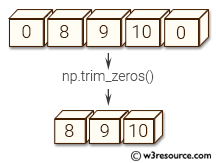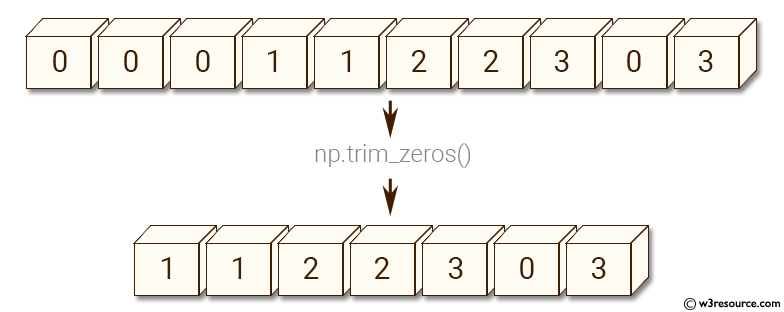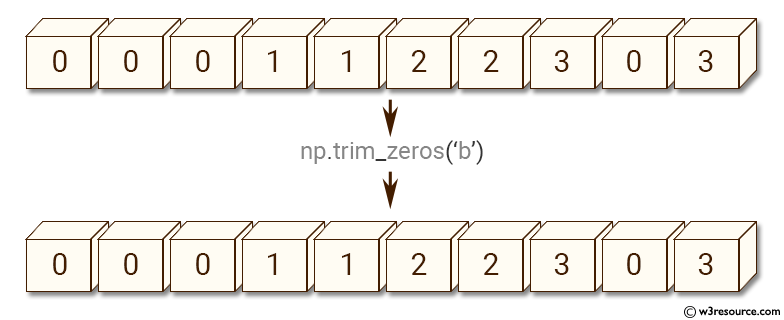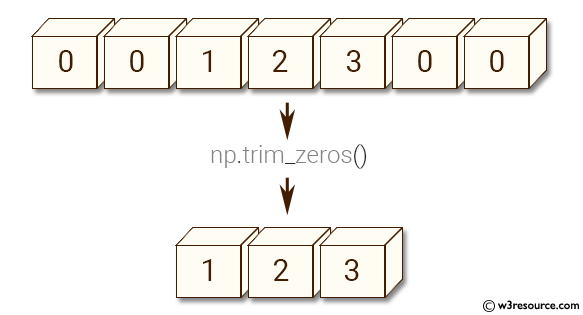# NumPy Array manipulation: trim_zeros() function

## numpy.trim_zeros() function

The trim_zeros() function is used to trim the leading and/or trailing zeros from a 1-D array or sequence.

Syntax:

`numpy.trim_zeros(filt, trim='fb')`Version: 1.15.0

Parameter:

Name Description Required /
Optional
filt Input array. Required
trim A string with 'f' representing trim from front and 'b' to trim from back. Default is 'fb', trim zeros from both front and back of the array. Optional

Return value:

trimmed : 1-D array or sequence The result of trimming the input. The input data type is preserved.

Example-1: numpy.trim_zeros() function

``````>>> import numpy as np
>>> a = np.array((0,0,0,1,1,2,2,3,0,3))
>>> np.trim_zeros(a)
array([1, 1, 2, 2, 3, 0, 3])
``````

Pictorial Presentation:Example-2: numpy.trim_zeros() function

``````>>> import numpy as np
>>> a = np.array((0,0,0,1,1,2,2,3,0,3))
>>> np.trim_zeros(a, 'b')
array([0, 0, 0, 1, 1, 2, 2, 3, 0, 3])
``````

Pictorial Presentation:Example-3: numpy.trim_zeros() function

``````>>> import numpy as np
>>> np.trim_zeros([0, 0, 1, 2, 3, 0, 0])
[1, 2, 3]
``````

Pictorial Presentation:Python - NumPy Code Editor:

Previous: resize()
Next: unique()

﻿